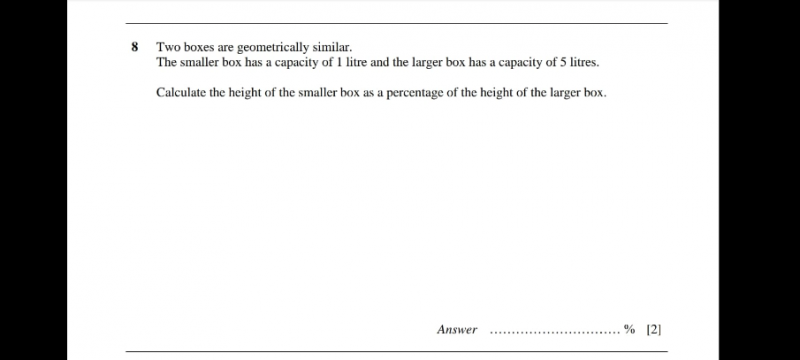# QuestionThank you .

Let h1 be the height of the small box

Let h2 be the height of the large box

If two solids are similar, the ratio of their volumes is equal to the cube of the ratio of their corresponding sides

(h2/h1) = (V2/V1) = 5/1

h2/h1 =  cube root (5)

h2 = cube root (5) h1

Therefore height of smaller box h1 as a percentage of height of bigger box, h2

= (h1 / cube root (5)h1)  x 100%  ~ 58.5%

0 Replies 1 Like